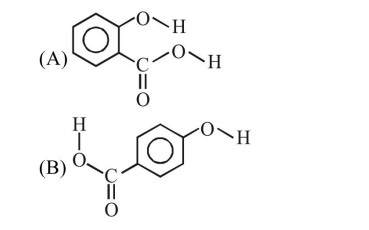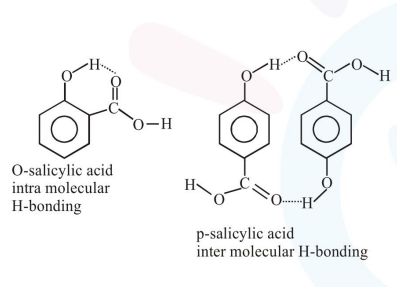# Consider the following molecules and statements related to them :

Question:

Consider the following molecules and statements related to them :(a) (B) is more likely to be crystalline than (A)

(b) (B) has higher boiling point than (A)

(c) (B) dissolves more readily than (A) in water

Identify the correct option from below :

1. only (a) is true

2. (a) and (c) are true

3. (b) and (c) are true

4. (a) and (b) are true

Correct Option: , 3

Solution:(a) B will be more crystalline due to more inter molecular interactions hence more efficient packing.

(b) B will have higher boiling point due to higher intermolecular interactions.

(c) $\mathrm{B}$ will be more soluble in water than $\mathrm{A}$ as B will have more extent of H-bonding in water So all three statements are correct

$\{$ Solubility date $\Rightarrow$ O-salicylic acid $=2 \mathrm{~g} / \mathrm{L}$

P-salicylic acid $=5 \mathrm{~g} / \mathrm{L}\}$